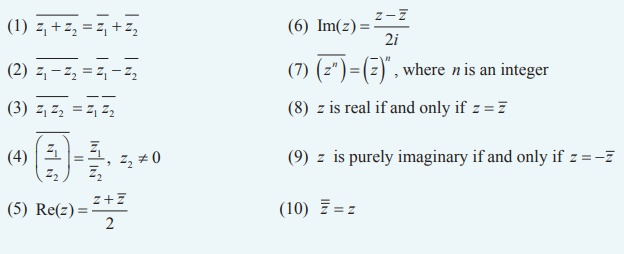Home | | Maths 12th Std | Summary

# Summary

Rectangular form of a complex number is x + iy (or x + yi) , where x and y are real numbers.

SUMMARY

In this chapter we studied

Rectangular form of a complex number is x + iy (or x + yi) , where x and y are real numbers.

Two complex numbers z1 = x1 + iy1 and z2 = x2 + iy2 are said to be equal if and only if

Re(z1 ) = Re(z2 )  and  Im(z1 ) = Im(z2 ) . That is  x1 = x2 and  y1 = y2 .

The conjugate of the complex number x + iy is defined as the complex number x - iy.

Properties of complex conjugatesIf  z = x + iy, then  ŌłÜ[x2 + y2] is called modulus of z . It is denoted by |z| .

Properties of Modulus of a complex numberFormula for finding square root of a complex numberLet r and ╬Ė be polar coordinates of the point P(x, y) that corresponds to a  non-zero complex number z = x + iy . The polar form or trigonometric form of a complex number P is

z = r(cos╬Ė + i sin╬Ė ) .

Properties of polar form

Property 1:

If z = r (cos╬Ė + i sin╬Ė ), then z-1 = 1/r  (cos╬Ė - i sin╬Ė ) .

Property 2:

If z1  = r1 (cos╬Ė1  + i sin╬Ė1 ) and  z2  = r2 (cos╬Ė2  + i sin╬Ė2 ),

then   z1 z2  = r1r2 (cos(╬Ė1  + ╬Ė2 ) + i sin(╬Ė1  + ╬Ė2 )) .

Property 3:

If  z1  = r1 (cos╬Ė1  + i sin╬Ė1 ) and  z2  = r2 (cos╬Ė2  + i sin╬Ė2 ) ,

Then z1/z2 = r1/r2 [cos(╬Ė1- ╬Ė2)  + i sin(╬Ė1- ╬Ė2) ]

de MoivreŌĆÖs Theorem

(a) Given any complex number cos ╬Ė + i sin ╬Ė and any integer n,

(cos╬Ė + i sin╬Ė )n = cos n╬Ė + i sin n╬Ė

(b) If x is rational, then  cos x ╬Ė + i sin x ╬Ė  in one of the values of (cos ╬Ė + i sin ╬Ė)x

The nth roots of complex number z = r (cos╬Ė + i sin╬Ė ) areTags : Complex Numbers , 12th Mathematics : UNIT 2 : Complex Numbers
Study Material, Lecturing Notes, Assignment, Reference, Wiki description explanation, brief detail
12th Mathematics : UNIT 2 : Complex Numbers : Summary | Complex Numbers